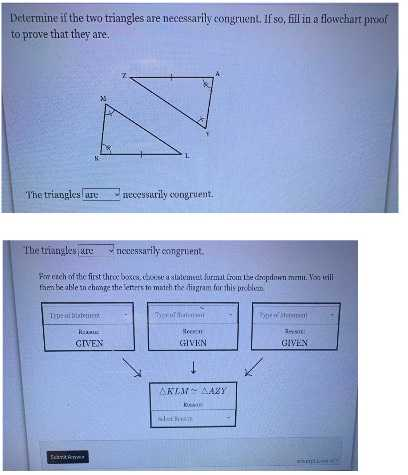### Still have math questions?$$\angle M= \angle Y\\\angle K= \angle A\\KL= AZ$$